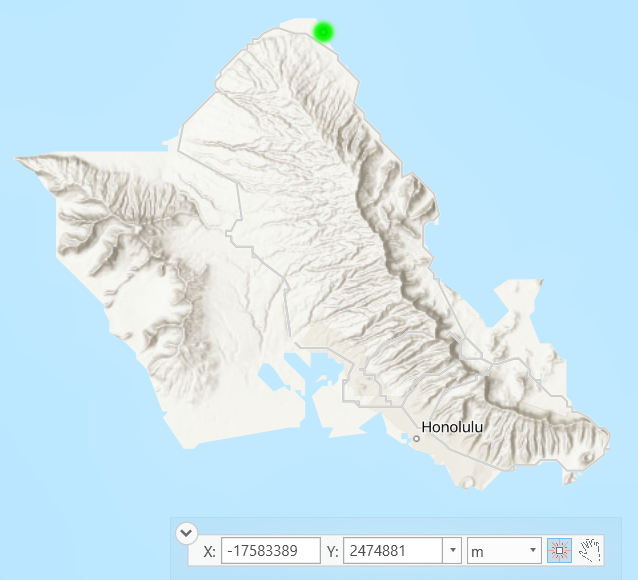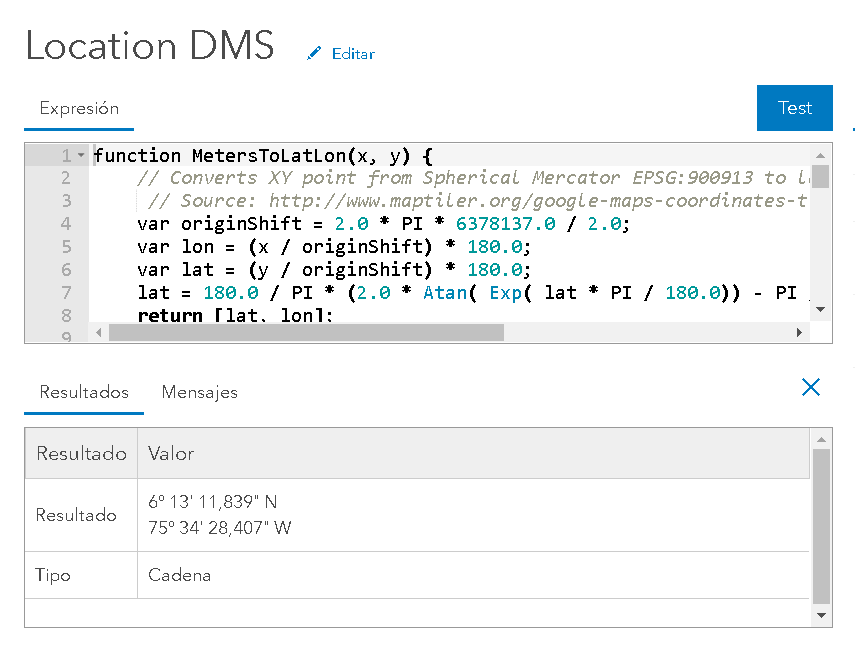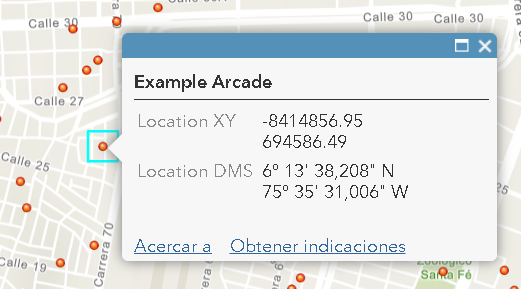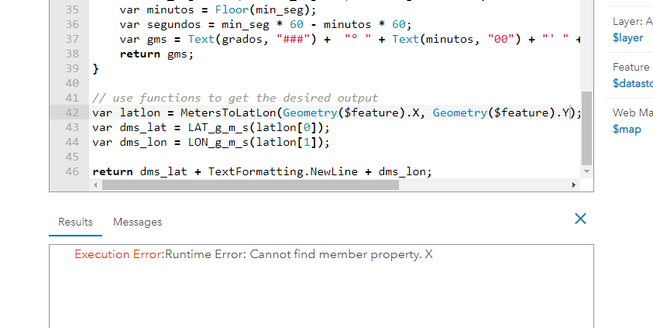# Converting geometry(\$feature) coordinates in a pop-up to decimal degrees?

3599
9
12-12-2019 02:02 PMby
New Contributor II

In arc gis online, I have a point feature map. When I create a new feature, I would like it to calculate the latitude and longitude in decimal degrees.

Right now, I am using the following code in the Arcade, but the coordinates are in the wrong format for my uses.

var geom = Geometry(\$feature);
return "(" + Round(geom.x, 3) + ", " + Round(geom.y, 3) + ")";

example of the returned data: (-17583389.106, 2474881.059)

What is the right expression for obtaining decimal degrees instead of what is provided above?

Tags (3)
1 Solution

Accepted SolutionsbyEsri Esteemed Contributor

Hi Dena S ,

You can do this and that would simplify the expression to something like this:

``````function MetersToLatLon(x, y) {
// Converts XY point from Spherical Mercator EPSG:900913 to lat/lon in WGS84 Datum
var originShift = 2.0 * PI * 6378137.0 / 2.0;
var lon = (x / originShift) * 180.0;
var lat = (y / originShift) * 180.0;
lat = 180.0 / PI * (2.0 * Atan( Exp( lat * PI / 180.0)) - PI / 2.0);
return [lat, lon];
}

// use functions to get the desired output
var latlon = MetersToLatLon(Geometry(\$feature).X, Geometry(\$feature).Y);
return Round(latlon,6) + TextFormatting.NewLine + Round(latlon, 6);‍‍‍‍‍‍‍‍‍‍‍‍‍``````
9 RepliesbyEsri Esteemed Contributor

Hi denars ,

I assume that you have Web Mercator Auxiliary Sphere coordinates in your web map and those should first be converted to WGS1984. An example how to do this:

``````function MetersToLatLon(x, y) {
// Converts XY point from Spherical Mercator EPSG:900913 to lat/lon in WGS84 Datum
var originShift = 2.0 * PI * 6378137.0 / 2.0;

var lon = (x / originShift) * 180.0;
var lat = (y / originShift) * 180.0;

lat = 180.0 / PI * (2.0 * Atan( Exp( lat * PI / 180.0)) - PI / 2.0);
return [lat, lon];
}

var latlon = MetersToLatLon(Geometry(\$feature).X, Geometry(\$feature).Y);‍‍‍‍‍‍‍‍‍‍‍‍‍‍``````

This will return lat and lon as decimal degrees. You can change the visualization by some more Arcade, like this:

``````Function LON_g_m_s(decimal_degrees) {
if (decimal_degrees < 0) {
} else {
}
var min_seg = Abs(decimal_degrees) * 60 - grados * 60;
var minutos = Floor(min_seg);
var segundos = min_seg * 60 - minutos * 60;
var gms = Text(grados, "###") +  "° " + Text(minutos, "00") + "' " + Text(segundos, "00.000") + '" ' + quad;
return gms;
}‍‍‍‍‍‍‍‍‍‍‍‍‍``````

and

``````Function LAT_g_m_s(decimal_degrees) {
if (decimal_degrees< 0) {
} else {
}
var min_seg = Abs(decimal_degrees) * 60 - grados * 60;
var minutos = Floor(min_seg);
var segundos = min_seg * 60 - minutos * 60;
var gms = Text(grados, "###") +  "° " + Text(minutos, "00") + "' " + Text(segundos, "00.000") + '" ' + quad;
return gms;
}‍‍‍‍‍‍‍‍‍‍‍‍‍``````by
New Contributor II

Thanks for the quick reply. I just started my first web map, how do I know what projection it is in?

Can you provide a little more guidance on how to apply this code? I added the first example into an attribute expression and saved it. This added a new field to my popup but without any associated coordinates. Thanks for the clarification!byEsri Esteemed Contributor

Hi Dena S ,

I assume that the coordinates are in Web Mercator Auxiliary Sphere,which is the default coordinate system in ArcGIS Online. Does the location correspond to the Northern part of O'ahu?If so, you can use the expression below to obtain a multiline text string containing the coordinates.First go into configuration of your pop-up. The scroll down to where you can define your Arcade expressions and add a new expression. Copy and paste the code below:

``````function MetersToLatLon(x, y) {
// Converts XY point from Spherical Mercator EPSG:900913 to lat/lon in WGS84 Datum
var originShift = 2.0 * PI * 6378137.0 / 2.0;
var lon = (x / originShift) * 180.0;
var lat = (y / originShift) * 180.0;
lat = 180.0 / PI * (2.0 * Atan( Exp( lat * PI / 180.0)) - PI / 2.0);
return [lat, lon];
}

Function LON_g_m_s(decimal_degrees) {
// convert decimal degrees longitud to DMS
if (decimal_degrees < 0) {
} else {
}
var min_seg = Abs(decimal_degrees) * 60 - grados * 60;
var minutos = Floor(min_seg);
var segundos = min_seg * 60 - minutos * 60;
var gms = Text(grados, "###") +  "° " + Text(minutos, "00") + "' " + Text(segundos, "00.000") + '" ' + quad;
return gms;
}

Function LAT_g_m_s(decimal_degrees) {
// convert decimal degrees latitud to DMS
if (decimal_degrees< 0) {
} else {
}
var min_seg = Abs(decimal_degrees) * 60 - grados * 60;
var minutos = Floor(min_seg);
var segundos = min_seg * 60 - minutos * 60;
var gms = Text(grados, "###") +  "° " + Text(minutos, "00") + "' " + Text(segundos, "00.000") + '" ' + quad;
return gms;
}

// use functions to get the desired output
var latlon = MetersToLatLon(Geometry(\$feature).X, Geometry(\$feature).Y);
var dms_lat = LAT_g_m_s(latlon);
var dms_lon = LON_g_m_s(latlon);

return dms_lat + TextFormatting.NewLine + dms_lon;‍‍‍‍‍‍‍‍‍‍‍‍‍‍‍‍‍‍‍‍‍‍‍‍‍‍‍‍‍‍‍‍‍‍‍‍‍‍‍‍‍‍‍‍‍‍``````

And test the expression (it should look something like this):Close the window and make sure that the expression is included in your attributes that will be shown in the pop-up (this is default behavior). In my case the result looks like this:by
New Contributor II

Thank you Xander Bakker‌!

This definitely worked.

How do I keep the coordinates in decimal degrees (21.69046, -157.962533) instead of converting to DMS (21°41'53.0"N 157°57'45.1"W)

Finally, is there documentation that describes the code that you used in the above expression, so that I can have a better understanding of how it works?

Many thanks!byEsri Esteemed Contributor

Hi Dena S ,

You can do this and that would simplify the expression to something like this:

``````function MetersToLatLon(x, y) {
// Converts XY point from Spherical Mercator EPSG:900913 to lat/lon in WGS84 Datum
var originShift = 2.0 * PI * 6378137.0 / 2.0;
var lon = (x / originShift) * 180.0;
var lat = (y / originShift) * 180.0;
lat = 180.0 / PI * (2.0 * Atan( Exp( lat * PI / 180.0)) - PI / 2.0);
return [lat, lon];
}

// use functions to get the desired output
var latlon = MetersToLatLon(Geometry(\$feature).X, Geometry(\$feature).Y);
return Round(latlon,6) + TextFormatting.NewLine + Round(latlon, 6);‍‍‍‍‍‍‍‍‍‍‍‍‍``````by
New Contributor II

This solution worked beautifully - thank you.

I want to push this web map to a publicly accessible web app. When I do this however, I don't see the coordinates showing up. For example, I tried publishing the map as an "edit" web app via configurable apps so that people can add locations to the map. However, when I do this, only the editable features show, and not expression features, such as coordinates. Is there a work around for this? Do I need to use a different kind of web app?

Thanks!byEsri Esteemed Contributor

Hi Dena S ,

As far as I know, the edit pop-up will only show the fields that can be edited (and Arcade based fields will not be editable). I still think that a normal edit (not in edit mode) should show the Arcade field. If it doesn't then you would be better off using a different template or a WAB with the edit widget, but which will show the pop-up you configured with the Arcade expression when you are not editing.New Contributor II

I can't thank you enough! I've been beating my head against a brick wall for days over this. Finally found your expression today, worked perfectly! Thank you! Thank you! Thank you!Occasional Contributor III

@XanderBakker I need the decimal degrees to appear like they do in your script, but when I copied and pasted it into my arcade expression builder in Map Viewer, it returns the following error that I don't understand: